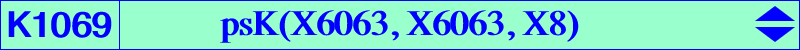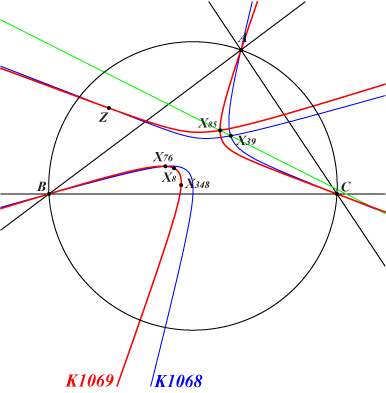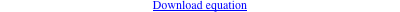too complicated to be written here. Click on the link to download a text file.X(8), X(76), X(85), X(348), X(22116) isotomic conjugates of X(173), X(268), X(3161), X(6600), X(8818) vertices of the cevian triangle of X(6063) = t X(55) see Z below Geometric properties :K1069 is the isotomic transform of K220 = psK(X55, X2, X6). See the related Q145. Z = a (a b + a c - b^2 - c^2) / (a^2 - b c) : : , SEARCH = - 0.816513116664244, lies on K1068 and K1069, on the lines {1,39}, {10,514}, {12,85}, etc. Z is now X(22116) in ETC (2018-09-04). Z is the transform of the common point X(659) of the trilinear polars of X(1) and X(83) under the mapping 𝚹 : P -> aQ described in page K1065.